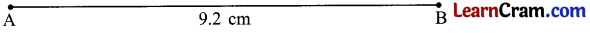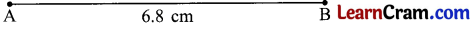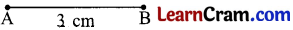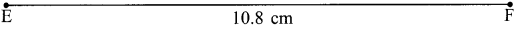# DAV Class 3 Maths Chapter 12 Worksheet 5 Solutions

The DAV Maths Book Class 3 Solutions and DAV Class 3 Maths Chapter 12 Worksheet 5 Solutions of Geometry offer comprehensive answers to textbook questions.

## DAV Class 3 Maths Ch 12 WS 5 Solutions

Question 1.
Draw the line segments of the following lengths and name them.

(a) 5 cm
Solution:(b) 7 cm
Solution:(c) 13 cm
Solution:(d) 4.5 cm
Solution:(e) 9.2 cm
Solution:(f) 6.8 cm
Solution:Question 2.
Draw the following line segments.

(a) AB = 3 cm
Solution:(b) XY = 9.1 cm
Solution:(c) MN = 7.3 cm
Solution:(d) EF = 10.8 cm
Solution:### DAV Class 3 Maths Chapter 12 Worksheet 5 Notes

Constructing line segments of given Length

Eg. Draw a line segment of length 4.6 cm

1. Place the ruler on page.
2. Mark with sharp pencil point A against zero mark of the ruler.
3. Mark second point ‘B’ against the mark representing 4 (big divisions) and 6 (sub¬divisions)
4. Join the points A and B.